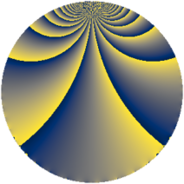Properties

 Label 448.1.lLevel $448$ Weight $1$ Character orbit 448.l Rep. character $\chi_{448}(209,\cdot)$ Character field $\Q(\zeta_{4})$ Dimension $2$ Newform subspaces $1$ Sturm bound $64$ Trace bound $0$

Related objects

Defining parameters

 Level: $$N$$ $$=$$ $$448 = 2^{6} \cdot 7$$ Weight: $$k$$ $$=$$ $$1$$ Character orbit: $$[\chi]$$ $$=$$ 448.l (of order $$4$$ and degree $$2$$) Character conductor: $$\operatorname{cond}(\chi)$$ $$=$$ $$112$$ Character field: $$\Q(i)$$ Newform subspaces: $$1$$ Sturm bound: $$64$$ Trace bound: $$0$$

Dimensions

The following table gives the dimensions of various subspaces of $$M_{1}(448, [\chi])$$.

Total New Old
Modular forms 24 6 18
Cusp forms 8 2 6
Eisenstein series 16 4 12

The following table gives the dimensions of subspaces with specified projective image type.

$$D_n$$ $$A_4$$ $$S_4$$ $$A_5$$
Dimension 2 0 0 0

Trace form

 $$2 q + O(q^{10})$$ $$2 q + 2 q^{11} - 2 q^{29} - 2 q^{37} - 2 q^{43} - 2 q^{49} + 2 q^{53} - 2 q^{63} - 2 q^{67} + 2 q^{77} - 2 q^{81} + 2 q^{99} + O(q^{100})$$

Decomposition of $$S_{1}^{\mathrm{new}}(448, [\chi])$$ into newform subspaces

Label Dim. $$A$$ Field Image CM RM Traces $q$-expansion
$a_{2}$ $a_{3}$ $a_{5}$ $a_{7}$
448.1.l.a $2$ $0.224$ $$\Q(\sqrt{-1})$$ $D_{4}$ $$\Q(\sqrt{-7})$$ None $$0$$ $$0$$ $$0$$ $$0$$ $$q-iq^{7}-iq^{9}+(1+i)q^{11}+iq^{25}+\cdots$$

Decomposition of $$S_{1}^{\mathrm{old}}(448, [\chi])$$ into lower level spaces

$$S_{1}^{\mathrm{old}}(448, [\chi]) \cong$$ $$S_{1}^{\mathrm{new}}(112, [\chi])$$$$^{\oplus 3}$$